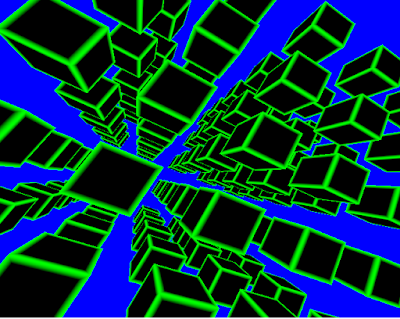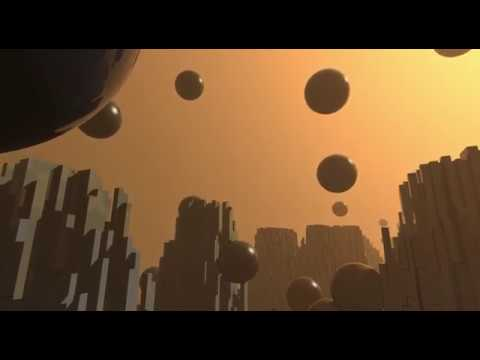## Monday, 17 June 2019

### Using Glium

I have recently spent some time with glium, the safe, native Rust wrapper for OpenGL. The library has pretty good tutorials on its website but I thought I log my experience with it as I get to grips with it and try build something non-trivial. ( At the moment I have a vague notion of combining some glium code with my earlier mod-player crate into something )

###It is very easy to set it up. All I need to do is to create a new rust project and add a glium dependency to it.
``````[dependencies]
glium = "*"
``````

### Setting up a window

The glium tutorials go into a fair bit of detail about how the display is setup and gradually introduces concepts such as the z-buffer. I am going jump straight into a screen setup that makes sense for most applications that do some 3D rendering.
Glium ( with glutin ) makes it very easy to setup an OpenGL window ready for rendering. The code below is all that is required to setup a rendering windows.
```use glium;
use glium::{glutin, Surface};

fn main() {
let mut events_loop = glutin::EventsLoop::new();
let wb = glutin::WindowBuilder::new();
let cb = glutin::ContextBuilder::new().with_depth_buffer(24);
let display = glium::Display::new(wb, cb, &events_loop).unwrap();

let mut closed = false;
while !closed {
let mut target = display.draw();
target.clear_color_and_depth((0.0, 0.0, 1.0, 1.0), 1.0);
target.finish().unwrap();

events_loop.poll_events(|ev| match ev {
glutin::Event::WindowEvent { event, .. } => match event {
glutin::WindowEvent::CloseRequested => closed = true,
_ => (),
},
_ => (),
});
}
}
```
The `events_loop` is a wrapper around the system's event queue. We need it to process events from the windowing system. Here the only event we care about is the event signalling the closing the window.
Glutin has a `WindowBuilder` for capturing all the window creation parameters. This is where we can set things like size, full-screen, title etc. The default parameters are fine for this program. Creating the `WindowBuilder` does not actually create the window but is an object that lets you create one.
The `ContextBuilder` is a builder for OpenGL contexts. All OpenGL calls are associated with a context that determines how the rendering is handled. The context controls things like; pixel format, depth buffer, stencil buffer, OpenGL version etc. Any information that controls how the rendering is performed and that needs to be known in advance goes into the context creation.
The only change to the defaults this program makes is to enable a 24-bit the depth buffer. ( I am using 24-bit depth buffer because many graphics cards would store the depth and stencil data into the same area allocating 24 bits for the depth and 8 bits for stencils. I am sticking by that convention here.)
The constructor of `glium::Display` takes the event loop and the contexts to create the actual window.
The code inside the while loop clears the display and checks for window close events. All OpenGL calls for rendering a frame have to be surrounded by code indicating the start and end of the graphics operations. In glium that is handled by the `Display::draw` and `Frame::finish()`
```        let mut target = display.draw();
// Rendering code goes here
target.finish().unwrap();
```
Having previously spent a lot of timne writing OpenGL code with 'raw' OpenGL calls, the Glium/glutin abstraction is excellent and really helps handle all the tedious boiler plate code.

Modern OpenGL requires that we set up shaders for the different stages of the rendering pipeline. At minimum this requires us to set up the vertex shader and the fragment shader. There are loads of excellent resources that explain how different shaders work (like https://learnopengl.com/)
My first vertex shader transforms the vertex from world position and applies a perspective projection matrix to it. In addition to transforming the position it also passes UV coordinates that can be used for texturing.
```    #version 140

in vec3 position;
in vec2 tex_coords;
out vec2 v_tex_position;

uniform mat4 matrix;
uniform mat4 perspective;
void main() {
v_tex_position = tex_coords;
gl_Position = perspective * matrix * vec4(position, 1.0);
}
```
The fragment shader is a also quite simple. It effectively creates a grid based on the texture coordinates passed into the shader. The nice thing about this shader is that it lets me see the rendering pipeline operating correctly without worrying about lighting or textures.
```    #version 140

in vec2 v_tex_position;
out vec4 color;

void main() {
float dst = min( v_tex_position.y, v_tex_position.x);
dst = min( dst, min( 1.0-v_tex_position.y, 1.0-v_tex_position.x) );

float intensity = smoothstep( 0.1, 0.0, dst );
vec3 col = vec3( 0.0, intensity, 0.0 );
color = vec4(col,1.0);
}
```
In Glium the shaders are setup using `Program::from_source` which takes all the shaders, compiles them and sets them up in a `Program` that can be used for rendering
``` let program =
.unwrap();
```

### Defining Vertex Structures

The vertex shader I created takes vertices with position and texture coordinates as its input so I need to create vertices with those features.
First I need to define the vertex structure. Glium and Rust make it very easy to define new it vertex types. The code below defines the vertex structure and auto-generates the code for the required traits.
```#[derive(Copy, Clone)]
struct Vertex3t2 {
position: [f32; 3],
tex_coords: [f32; 2],
}
glium::implement_vertex!(Vertex3t2, position, tex_coords);
```

### Using nalgebra-glm

When I started working with glium I was suprised to find that it didn't come with code for handling vectors and matrices. Initially I just used wrote up my own code but this became quite tedious and error-prone. This is when I discovered the excellent nalgebra-glm crate. Including the crate in my project made the everything much easier. I recommend using it ( or any other decent matrix math crate ) when working with OpenGL.
``````nalgebra-glm = "0.4.0"
``````

### Creating the objects

Creating objects for display requires vertex buffers and index buffers
• Index buffers control how the vertices are connected to form triangles.
• Vertex buffers define the actual vertices and any data that belongs to them ( like texture coordinates )
I am using triangle lists for my index information. This means that each set of 3 vertices defines a triangle. Because triangles in a triangle list do not share vertices this is quite an inefficient way to use vertices. It is also very simple because it doesn't require any indices. Essentially we create an index buffer that has no indices.
```    let indices = glium::index::NoIndices(glium::index::PrimitiveType::TrianglesList);
```
The vertex buffers are created by passing glium an array of vertices. For this project I want to create some cubes to display. I break this down by first creating a function for creating a quad. Once I have the quad function I can use it to create the cube.
Because I want to reuse the quad for all the faces I define the quad by its bottom-left vertex and vectors pointing up and to the right. This means that I need to know whether I am working with a left or right handed coordinate system. Whilst writing the code for the cube builder I had to repeatedly check that I got the handedness the right way around.
```fn add_quad(dest: &mut Vec<Vertex3t2>, bottom_left: glm::Vec3, up: glm::Vec3, right: glm::Vec3) {
let top_left: glm::Vec3 = (bottom_left + up).into();
let top_right: glm::Vec3 = (top_left + right).into();
let bottom_right: glm::Vec3 = (bottom_left + right).into();
dest.push(Vertex3t2 {
position: bottom_left.into(),
tex_coords: [0.0, 0.0],
});
dest.push(Vertex3t2 {
position: top_left.into(),
tex_coords: [0.0, 1.0],
});
dest.push(Vertex3t2 {
position: top_right.into(),
tex_coords: [1.0, 1.0],
});
```
The above takes a reference to the vector where the quad vertices are stored, calculates the positions for the quad corners and pushes the vertices into the vector and output one triangle. To complete the quad I output three more vertices.
```    dest.push(Vertex3t2 {
position: bottom_left.into(),
tex_coords: [0.0, 0.0],
});
dest.push(Vertex3t2 {
position: top_right.into(),
tex_coords: [1.0, 1.0],
});
dest.push(Vertex3t2 {
position: bottom_right.into(),
tex_coords: [1.0, 0.0],
});
```
I need to be careful to make sure the triangle vertices are output in the same winding order. If I float above the visible side of the triangle and look down on it all the points should go in a clockwise order. This will allow me to use backface culling to reduce the number of rasterized triangles. It will also make it easy to generate normals for all the triangle vertices if they have the same winding order.
Once I have function for generating the quad I can use it generate a cube. A cube is just six quads with different orientations.
```fn add_cube(verts: &mut Vec<Vertex3t2>, pos: &glm::Vec3) {
verts,
glm::vec3(-0.5, -0.5, 0.5) + pos,
glm::vec3(0.0, 1.0, 0.0),
glm::vec3(1.0, 0.0, 0.0),
);
//..5 more sides
```
...the cube generator lets me generate an array of cubes.
```    let mut verts: Vec<Vertex3t2> = Vec::new();
for x in -2..3 {
for y in -2..3 {
for z in -2..3 {
&mut verts,
&glm::Vec3::new(x as f32 * 2.0, y as f32 * 2.0, z as f32 * 2.0),
);
}
}
}
```
Once I have generated all the vertices glium can turn them into a vertex buffer object that can be used for rendering.
```    let vertex_buffer = glium::VertexBuffer::new(&display, &verts).unwrap();
```

### Setting up matrices

Before we can render the object we need to setup the world and perspective matrices. As discussed, I use nalgebra-glm for all matrix calculations.
```        let mut model_view = glm::rotate_z(&glm::identity(), t);
model_view = glm::translate(&model_view, &glm::vec3(0.0, 0.0, -12.0));
model_view = glm::rotate_x(&model_view, t / 2.0);
model_view = glm::rotate_y(&model_view, t / 2.0);
let view: [[f32; 4]; 4] = model_view.into();
```
The first four lines create an identity matrix and apply translation and rotation to it. The last line copies the matrix from a matrix structure into a 4*4 `f32` array which can be sent to OpenGL. The type of `view` must be defined so `into` knows what type the matrix needs to be converted into.
GLM has a helper function `glm::perspective` for creating the perspective matrix;
```        let perspective = glm::perspective(1.0, 3.14 / 2.0, 0.1, 1000.0);
let p: [[f32; 4]; 4] = perspective.into();
```
This create a projection matrix that is ~90 degrees wide ( the field of view argument is passed in radians. 3.14 / 2.0 ) with a minimum and maximum depth of 0.1 and 1000.
Both of the matrices are passed to OpenGL in a uniform block. Uniform is data that is passed into the shader and every instance of the rendered seems the same version of the data.
Glium has a `glium::uniform!` macro that create a uniform block from the data passed to it. The names of the fields in the uniform macro must match the names of the uniform variables in the shaders. This is how glium binds the uniform data to the shaders.
To create the uniform block we just use the macro.
```    let uniforms = glium::uniform! {  matrix: view, perspective: p };
```

### Drawing the object with the right parameters

The final structure we need before we calling the draw function is a `glium::DrawParameters`. Glium uses this to tell OpenGL about things like culling and z-buffering. Glium does provide a default `DrawParameters` block bit does not enable the z-buffer so we need to setup one.
```    let params = glium::DrawParameters {
depth: glium::Depth {
test: glium::draw_parameters::DepthTest::IfLess,
write: true,
..Default::default()
},
backface_culling: glium::draw_parameters::BackfaceCullingMode::CullCounterClockwise,
..Default::default()
};
```
This sets up a `DrawParameters` structure with defaults except for enabling z-buffer writes, setting the test type and enabling the backface culling. I find the strange `..` for using the defaults a bit odd and have not found any good documentation on this syntax.
We know have everything in place to draw the object.
```        target.draw( &vertex_buffer, &indices, &program, &uniforms, &params ).unwrap();
```

### Conclusion

So far glium has been very pleasant to work with, striking just the right balance between hiding complexity and getting out of the way when it is not needed. I will definitely continue to use it for my projects.
The code in this project is very simple as it does not make use of instancing or have any kind of lighting model. This is something I want to look at next.